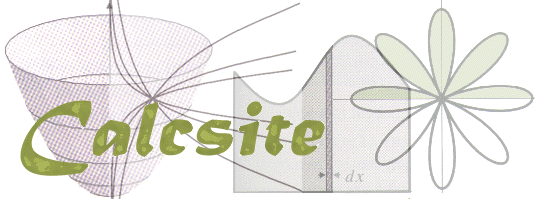The purpose of Calcsite is to provide an introduction to calculus--its methods, techniques, and modeling capabilities. If you want to learn what calculus is all about, this is the place.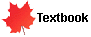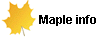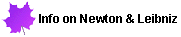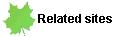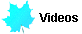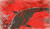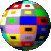Math 3 Math Dept